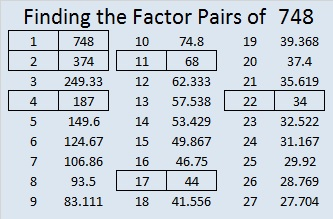# 748 and Level 2

• 748 is a composite number.
• Prime factorization: 748 = 2 x 2 x 11 x 17, which can be written 748 = (2^2) x 11 x 17
• The exponents in the prime factorization are 2, 1, and 1. Adding one to each and multiplying we get (2 + 1)(1 + 1)(1 + 1) = 3 x 2 x 2 = 12. Therefore 748 has exactly 12 factors.
• Factors of 748: 1, 2, 4, 11, 17, 22, 34, 44, 68, 187, 374, 748
• Factor pairs: 748 = 1 x 748, 2 x 374, 4 x 187, 11 x 68, 17 x 44, or 22 x 34
• Taking the factor pair with the largest square number factor, we get √748 = (√4)(√187) = 2√187 ≈ 27.34958866.Here is today’s puzzle:Print the puzzles or type the solution on this excel file: 10 Factors 2016-01-18

—————————————

Here is some more about composite number 748:

748 can be written as the sum of consecutive numbers 3 ways:

• 90 + 91 + 92 + 93 + 94 + 95 + 96 + 97 = 748; that’s 8 consecutive numbers.
• 63 + 64 + 65 + 66 + 67 + 68 + 69 + 70 + 71 + 72 + 73 = 748; that’s 11 consecutive numbers.
• 36 + 37 + 38 + 39 + 40 + 41 + 42 + 43 + 44 + 45 + 46 + 47 + 48 + 49 + 50 + 51 + 52 = 748; that’s 17 consecutive numbers.

Because 17 is one of its factors, 748 is the hypotenuse of Pythagorean triple 352-660-748, and 352² + 660² = 748².

748 is the sum of 3 squares two different ways. Both ways contain a duplicate square.

• 26² + 6² + 6² = 748
• 18² + 18² + 10² = 748

748 is palindrome MM in BASE 33 (M = 22 base 10); note that 22(33) + 22(1) = 748.

—————————————This site uses Akismet to reduce spam. Learn how your comment data is processed.